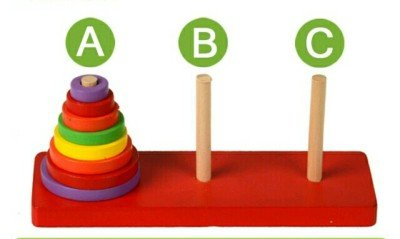# 递归的概念

• 递归函数
• 举例说明，例如Fabonacci, Ackerman
• 递归函数的算法设计
• 递归过程
• 例如Hanoi问题
• 递归过程的算法设计
• 递归算法的复杂都分析
• 复杂度函数推导
• 复杂度渐进阶计算
• 递归算法的调用机制

int fibonacci(int n){
if(n <= 1)
return 1;
return fibonacci(n-1)+fibonacci(n-2)
}


Hanoi 塔问题。古代有一个梵塔，塔内有三个座 A、B、C，A 座上有 64 个盘子，盘子大小不等，大的在下，小的在上。有一个和尚想把这 64 个盘子从 A 座移到 B 座，但每次只能允许移动一个盘子，并且在移动过程中，3 个座上的盘子始终保持大盘在下，小盘在上。如下图void hanoi(int n, int a, int b, int c){
if(n>0){
hanoi(n-1, a, c, b);
move(a,b);
//可以是 prinf("把 %d 从 %d 移到 %d", n, a, b)
hanoi(n-1, c, b, a);
}
}


1. 将实参指针、返回地址等传送给被调用函数
2. 为被调用的函数分配存储区
3. 将控制转移指向被调用函数的入口
4. 被调用函数执行，并保存计算结果
5. 释放分配的存储区
6. 将控制转移指向原函数

$$\begin{cases} P(R)=\sum_{k=1}^n a_kP(R-a_k)\\ P(a_k)=a_k \end{cases}$$

# 分治法的基本思想

• 分治算法的基本思想是什么？
• 分治算法的基本框架(三个步骤)
• 问题分解
• 子问题递归求解(初始情形求解)
• 子问题解的合并
• 分治问题算法伪代码描述
• 分治问题算法的递归实现
• 分治问题算法的复杂度分析
• T(n) = 分解的复杂度 + 子问题复杂度 + 解的合并复杂度

divide-and-conquer(P){
if(|P|<=n0){
solve(P);
}
divide P into P1, P2, …, Pk;
for(i=1;i<=k;i++)
yi = divide-and-conquer(Pi);
return merge(y1, y2, …, yk);
}